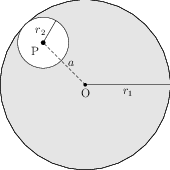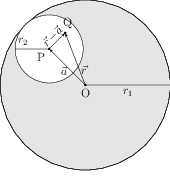# Gauss's Law and its Applications

By Gauss's law, the flux of electric field $\vec{E}$ through a closed surface $S$ is equal to the algebraic sum of the charges enclosed by this surface, divided by $\epsilon_0$ i.e., \begin{align} \oint \vec{E}\cdot\mathrm{d}\vec{S}=\frac{q_{enc}}{\epsilon_0} \end{align}

## Problems from IIT JEE

Problem (IIT JEE 2015):Consider a uniform spherical charge distribution of radius $r_1$ centered at the origin O. In this distribution, a spherical cavity of radius $r_2$, centered at P with distance $\mathrm{OP}=a=r_1-r_2$ (see figure) is made. If the electric field inside the cavity at position $\vec{r}$ is $\vec{E}(\vec{r})$, then the correct statement(s) is(are),

1. $\vec{E}$ is uniform, its magnitude is independent of $r_2$ but its direction depends on $\vec{r}$.
2. $\vec{E}$ is uniform, its magnitude depends on $r_2$ and its direction depends on $\vec{r}$.
3. $\vec{E}$ is uniform, its magnitude is independent of $a$ but its direction depends on $\vec{a}$.
4. $\vec{E}$ is uniform and both its magnitude and direction depend on $\vec{a}$.

Solution:Let $\rho$ be the charge density of the spherical charge distribution of radius $r_1$ centred at the origin O. A spherical cavity of radius $r_2$ centred at P with distance $\mathrm{OP}=a=r_1-r_2$ is made in the spherical distribution (see figure). The sphere with cavity is equivalent to a sphere of uniform charge density $-\rho$ and radius $r_2$ centred at P embedded in the original sphere. Thus, electric field at a point Q in the cavity is superposition of (i) electric field at Q due to the sphere of charge density $\rho$ and radius $r_1$ centred at O (say $\vec{E}_1$), and (ii) electric field at Q due to the sphere of charge density $-\rho$ and radius $r_2$ centred at P (say $\vec{E}_2$). Let $\vec{a}$, $\vec{r}$, and $\vec{r}-\vec{a}$ be the vectors as shown in the figure. The electric fields $\vec{E}_1$, $\vec{E}_2$, and their superposition $\vec{E}_{12}$ are given by, \begin{align} &\vec{E}_1=\frac{1}{4\pi\epsilon_0}\frac{\frac{4}{3}\pi |\vec{r}|^3\rho}{|\vec{r}|^2}\hat{r}=\frac{\rho}{3\epsilon_0}\,\vec{r},\\ &\vec{E}_2=-\frac{\rho}{3\epsilon_0}\,(\vec{r}-\vec{a}),\\ &\vec{E}_{12}=\vec{E}_1+\vec{E}_2=\frac{\rho}{3\epsilon_0}\,\vec{a}. \end{align} Thus, electric field at a point within the cavity is uniform and both its magnitude and direction depend on $\vec{a}$. The readers are encouraged to draw the electric lines of forces both inside and outside the cavity.

## Some Applications of Gauss's Law

Example 1: Suppose that we have in vacuum a system of fixed point charges in equilibrium. Let us consider one of these charges, e.g. a charge $q$. Can its equilibrium be stable? The answer is no.

Example 2: The electric field of a uniformly charged plane sheet with surface charge density $\sigma$? The answer is $\vec{e}=\frac{\sigma}{2\epsilon_0}\hat{n}$.

Example 3: The electric field of two parallel planes charged uniformly with densities $\sigma$ and $-\sigma$? The field in space between two planes is $\vec{e}=\frac{\sigma}{\epsilon_0}\hat{n}$ and it is zero outside this space.

Example 4: The electric field of an infinite circular cylinder uniformly charged over the surface so that the charge $\lambda$ corresponds to its unit length? The field is given by $\vec{E}=\frac{\lambda}{2\pi\epsilon_0}\hat{r}$.

Example 5: The electric field of a spherical surface uniformly charged by the charge $q$? The field is given by $\vec{E}=\frac{q}{4\pi\epsilon_0 r^2}\hat{r}$ for points outside the sphere and $\vec{E}=\frac{q r}{4\pi\epsilon_0 a^3}\hat{r}$, where $a$ is radius of the sphere.

## Problems from Concepts of Physics

Problem: (COP Volume 2 Chapter 30 Exercise 17): A nonconducting sheet of large surface area and thickness $d$ contains uniform charge distribution of density $\rho$. Find the electric field at a point $P$ inside the plate at a distance $x$ from the central plane. Draw a qualitative graph of $E$ agains $x$ for $0 Solution: (Download pdf)Since surface area of the sheet is large, we can assume this to be an infinite sheet. By symmetry, the direction of the electric field inside (as well as outside) the sheet is perpendicular to its surface and is in outward direction (if$\rho$is positive). Also, the field at central plane should be zero. Consider a cylinder of length$x$, cross-section area$A$($=\pi r^2$) and one of its face located on the central plane (see middle figure) as the Gaussian surface. The flux through the curved surface of this cylinder is zero because field is perpendicular to the area element on the curved surface i.e.,$\mathrm{d}\phi=\vec{E}\cdot \mathrm{d}\vec{A}=|\vec{E}| |\mathrm{d}\vec{A}|\cos90=0$. The flux through the face on the central plane is zero because$E=0$on the central plane. The flux through the other face is$E_\text{in}A$, where$E_\text{in}$is the field inside the plate at a distance$x$from the central plane. Thus, total flux through this Gaussian surface is$\phi=E_\text{in} A$. The charge enclosed in this Gaussian surface is$q_{en}=\rho V= \rho A x$. Thus, by Gauss's law,$\phi=q_{en}/\epsilon$i.e.,$E_\text{in}A=\rho A x/\epsilon$which gives$E_\text{in}=\rho x/\epsilon$(here$\epsilon$is the permittivity of the insulator). For field outside the plate, consider a similar cylinder but of length$x>d$. The flux through this cylinder is$\phi=E_\text{out} A$and charge enclosed in this is$q_\text{en}=\rho A d$. Apply Gauss's law to get$E_\text{out}=\rho d/\epsilon_0$. Note that the field outside is independent of$x$i.e., it is a constant. Here$\epsilon_0$is permittivity of the free space. Note that field is not continuous at$x=d$(because$\epsilon\neq \epsilon_0\$). Generally, field on the boundary of charged insulators is dis-continuous.

This problem can be solved using different Gaussian surfaces. The readers are encouraged to find the field by using other Gaussian surfaces shown in the figure.